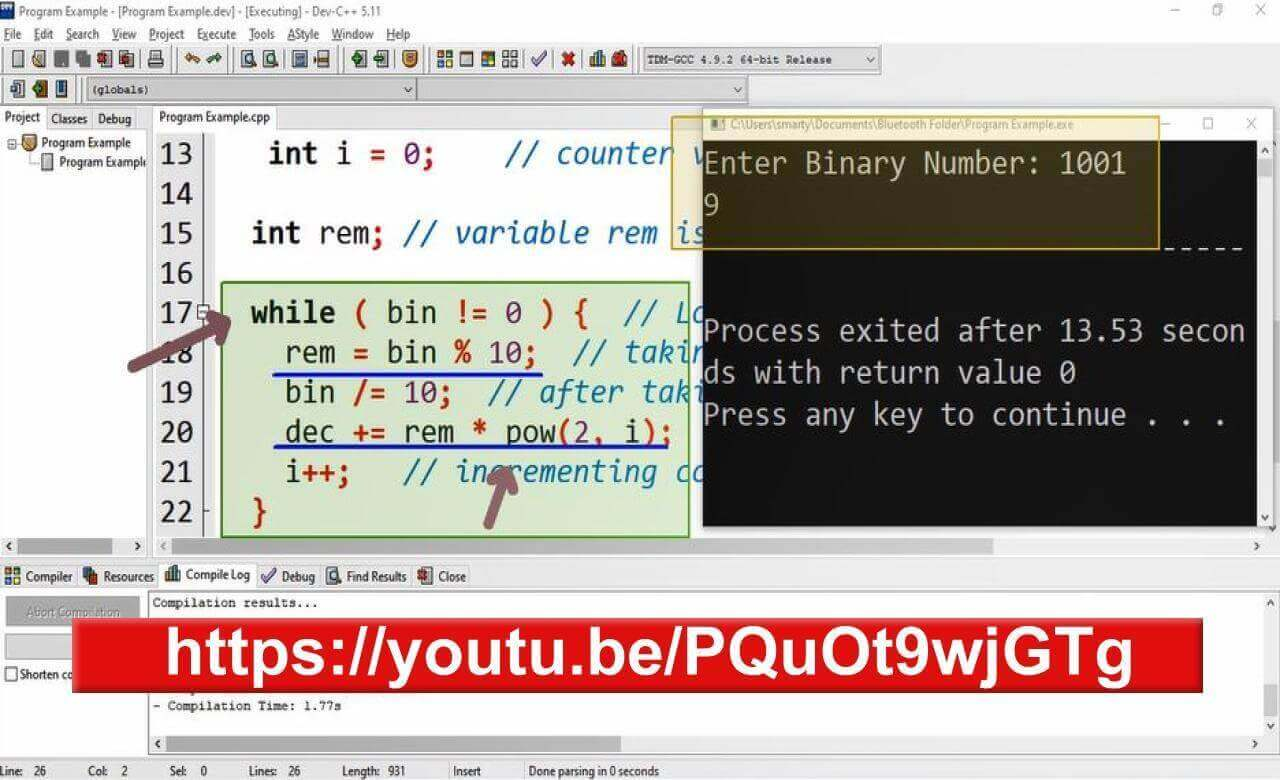# Program To Convet Binary Number Into Decimal In Cpp

## Source Code

#include <iostream>
#include<cmath>
// Library file included for using pow() function

using namespace std;  // standar name space.

int main(int argc, char** argv) {
long int bin;   // variable is declared to get binary input

cout<<"Enter Binary Number: ";
cin>>bin;   // taking input in variable

int dec = 0// variable to store resultant decimal value

int i = 0;    // counter variable

int rem; // variable rem is used to temporarily store remainders in loop.

while ( bin != 0 ) {  // Loop until input number goes to 0
rem = bin % 10;  // taking remainder of number. This will provide last digit
bin /= 10;  // after taking last digit reducing number by last digit.
dec += rem * pow(2, i);  // taking power of last digit by its position
i++;   // incrementing counter variable to next position
}

cout<<dec;  // printing calculated decimal number
return 0;
}

## Output